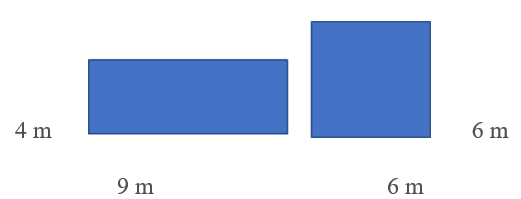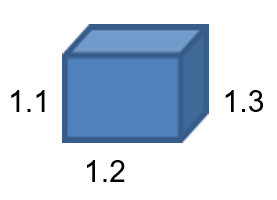Mathematics
The 3 Means: Arithmetic Mean, Geometric Mean, Harmonic Mean
9k views

 1 Introduction 2 Arithmetic Mean 3 Geometric Mean 4 Harmonic Mean 5 Relationship between Arithmetic Mean and Geometric mean 6 Summary 7 External References

25 September 2020

## Introduction

The word Mean comes from the French word “meien”.

Just to recap Mean is the average or the ratio of the sum of all given observations to the total no. of observations.Let's say $$x_1, x_2, x_3, x_4$$ are our observations then the mean denoted as X bar $$= x_1+ x_2+x_3+ x_4.$$ Or we can write as as

$\overline{\text{X}}=\Sigma \frac{\rm{X}}{\rm{n}}$

There are 3 types of mean that are Arithmetic Mean, Geometric Mean and Harmonic mean that we will discuss in detail.

Here are some additional points that talk about how to use all three means in math. To view them click on the Download button.

## What is the Arithmetic Mean of numbers?

If a, b and c are three terms given to be in A.P. then using the property of A.P. we get

$$b – a = c – b$$

Rearranging the terms,

$$2b = a + c$$

and this b is called the Arithmetic Mean between a and c.

Let’s understand Arithmetic Mean with an example: -

We have to find the average height of professional cricket players using the following samples:

 Players Height (m) X1 1.90 X2 2.00 X3 2.10 X4 2.15 X5 1.80

\begin{align}\overline{\text{X}}&=\frac{(1.90 + 2.00 + 2.10+ 2.15 + 1.80)}{5}\\&= 1.99 \,\text{m which is actually very tall.}\end{align}

So, the Arithmetic mean is actually the sum of all observations divided by no. of observations.

$\overline{\text{X}}=\Sigma \frac{\rm{X}}{\rm{n}}\quad \text{ (Arithmetic Mean)}$

## What do you mean by Geometric Mean?

In mathematics, the geometric mean is a mean, which specifies the central tendency of a set of numbers by using the multiply of their values.

The general form of a GP is x, xr, xr2, xr3 and so on.

Therefore $$\text{G.P Mean }= n\sqrt{ \pi r}$$

Here $$\pi$$ symbol pie would mean multiply all the elements of r.

Geometric Mean is unlike Arithmetic mean wherein we multiply all the observations in the sample and then take the nth root of the product.

Let’s understand this a bit more with examples. Geometric Mean gets its name from Geometry.

Let’s say a rectangle has a length 9m and a breadth as 4m then with the given dimension and equivalent area what is the length of a square?So here first we find out the area of the rectangle which is $$9 \times 4 = 36\text{ m.}$$

In this case, just from our knowledge, we can say that for a square the side length would be $$6\text{ m} \times 6 \text{ m}.$$ But if we apply the formulae of G.P

Then we get Square side length $$= \sqrt{9} \times 6 = \sqrt{36} = 6\text{ m}$$

So, this is where Geometric Mean gets its name.

 Example 2

I invested Rs. 100/- in Reliance stock which gained 10% in the 1st Year, 20% in the 2nd Year, and 30% in the 3rd Year. What is the average yearly rate of return?

Solution:

So, look at the below table of my investment and the value of my investment in subsequent years.

 Year Value of Investment (Rs.) Year 0 100 Year 1 110 Year 2 132 Year 3 171.60

Referring to the above table if I have to gain 10% on my investment in that 1st Year, it will give me Rs. 110/-,

Now to gain 20% in 2nd year I get 20% of 110 i.e. Rs. 132 and to gain 30% in 3rd year I get 30% of 132 i.e. Rs.171.60.

But actually, if you look at, I have just multiplied 1.1 in the 1st Instance,1.2 in the second instance, and 1.3 in the 3rd instance.

So, you can see here we have changing the yearly rate of return of the 3 years.

But here we are trying to see if we can find the yearly rate of return which is the same for each of these 3 years that gets us the same value for my investment in the 3rd year i.e. Rs. 171.60?

Now the reason I say it is a Geometrical problem is that imagine it as a 3-dimensional rectangular cube with side length as $$1.1,1.2,1.3$$ respectively.

We can ask what is the side length of the square-cube with the same volume?So even though it’s a financial question there is a geometrical analogy which is why we use geometric mean.

Hence, you see if we can multiply $$1.1 \times 1.2 \times 1.3$$ we get $$1.716$$ which when multiplied with my investment in the year 0, it gives us $$171.6.$$ This is my total value of the the investment in the 3rd year.

So now we take the cube root of $$1.716$$ that will give us an effective average of the yearly rate of return.

Again, if we apply for the above numbers in the Geometric Mean formula, we get

\begin{align}3\sqrt{1.716=1.1972}\end{align}

So, in order words, if we have to get Rs.171.60 from Rs. 100 in 3 years we can multiply $$1.1972$$ three times that gives us an average yearly rate of return as 19.72%

So, to summarize,

$\text{Geometric Mean}=\left[(1+r_1)+(1+r_2)\rm{x} \dots \rm{x}(1+r_n)\right]^{\frac{1}{n}-1}$

Similarly, If a, b, and c are three terms given to be in G.P. then using the property of G.P. we get

$\frac{b}{a}=\frac{c}{b}$

Rearranging the terms we get

$b^2=ac$

And $$b=\ \sqrt{ac}$$

## What is the Harmonic Mean of numbers?

### What is harmonic mean?

For any two terms ‘a’ and ‘b’, $$\text{Harmonic Mean} = \frac{(2 a b)}{ (a + b)}$$

Let’s first understand where does the word or concept of Harmonic comes from? Harmonic mean is clearly a part of a harmonic series.

Harmonic comes from musical word harmony.

### How to Find Harmonic mean?

Continuing on this example to figure out how to find harmonic mean we go through the process given below.

So, let’s say whenever we have a particular musical note for example say x at a frequency of 110 Hz and we strike one particular string then not only we get one 110 Hz sound wave is emanated but there are other harmonic overtones like 220 Hz 330 Hz,440 Hz,550 Hz…etc is emanated.

That means along with 110 Hz frequency we get sound waves of a little bit of each of the other-mentioned harmonic overtones.

We are not going into musicality but we know as per science wavelength is proportionate to the inverse of frequency.So, let’s say for wavelength 1st frequency is 1 then for a second it will be $$\frac{1}{2}$$ of the original and for 3rd frequency, the wavelength will be $$\frac{1}{3}$$ of the original.

It means this wavelength is the harmonic series.

So, from the below table we can say the harmonic mean of $$\frac{1}{2}$$ and $$\frac{1}{4}$$ is $$\frac{1}{3}$$.

It is because of this inverting that happens between frequency and wavelength. To define the average of two particular wavelengths we need to find the Harmonic average or the Harmonic mean.

 Frequency Wavelength 110 1 220 1/2 330 1/3 440 1/4 550 1/5

Harmonic mean is mainly used when we are dealing with the rate of change or average of rate is desired like average speed.

The harmonic means for n data values, assuming no data value is 0, is given by the equation below.

So similar method can be used whenever the question arises that “how to find harmonic mean?”The Harmonic mean is calculated as n divided by reciprocals of rates (r). Below formulae is used for Harmonic mean H, n is the no. of observations.

\begin{align}\rm{H}= \frac{n}{\frac{1}{r}+\frac{1}{r_2}+\frac{1}{r_3}+\dots\frac{1}{r_3}}\quad \text{ or } \quad \rm{H}=\frac{n}{\Sigma{\frac{1}{r}}} \end{align}

Note: we can take LCM of the denominator that is (r) in the case of Individual series

Or we can calculate the reciprocal of the rates using the M+ functionality of the calculator.

 Example:-

Let me ask you what is my average speed if I swim in the first 5 min. at 15km/hr and another 5 min. at 10km/hr.

This you can easily answer is $$12.5 \text{ km/hr}$$ by simply finding the Average of fixed time i.e. using average speed formulae of Distance /Time or Arithmetic mean that is adding things up $$(15+10)$$ divided by n which in this case is $$n = 2.$$

Distance/Speed 🡪 Average Across Fixed Time 🡪 Arithmetic Mean

But what if I ask what is my average speed If I swim first one lap at $$15\text{ km/hr }$$and the 2nd lap at $$10 \text{ km/hr?}$$

This no longer just an Arithmetic mean because my first 1 lap @ 15km/hr can be done faster than 2nd lap @ 10km/ hr so if you observe I can spend more time in swimming 2nd lap then the 1st. lap.

So here we cannot just say that my average speed is 12.5 km/hr.

It has to be the harmonic mean of both 15 km/hr and 10km/hr as we have to find average across fixed distance which is expressed as a rate rather than average across fixed time.

Distance/Speed 🡪 Average Across Fixed Distance 🡪 Harmonic Mean

\begin{align}\rm{H} &= \frac{2}{(\frac{1}{15}+\frac{1}{10})} \\\\&=\frac{2}{(\frac{25}{150})} =\frac{2}{(\frac{1}{6})} \\\\&= 12\end{align}

Therefore, my average speed is 12km/hr.

If you notice 12km/hr is closer to 15km/hr and 10 km/hr which is expected as I am swimming slightly longer in 2nd lap rather than 1st lap because of the fixed distance

Imp Note:

• If all observations are the same then, the Harmonic mean is equal to a single observation
• The harmonic mean is not affected by the change of origin

## Relationship between Arithmetic Mean and Geometric mean?

Let A and G be A.M. and G.M.

So,

$A=\frac{a+b}{2} \text { and } G=\sqrt{ab}$

Now let’s subtract the two equations

\begin{align}A-G&=\frac{a+b}{2}-\sqrt{a b} \\\\&=\frac{a+b-2 \sqrt{a b}}{2} \\\\&=\frac{(\sqrt{a}-\sqrt{b})^{2}}{2} \geq 0\end{align}

This indicates that $$A ≥ G$$

## Summary

To summarize the article in a short concise manner, here is the list of formulas of all the three means:

$$\overline{\text{X}}=\Sigma \frac{\rm{X}}{\rm{n}}$$ Arithmetic Mean

$$b=\sqrt{ac}$$ Geometric Mean

$$\rm{H}=\frac{n}{\Sigma \frac {1}{r}}$$ Harmonic Mean

And the relationship between AM and GM is as follows,

$A ≥ G$

Examples were solved to get an idea of how to find arithmetic mean, how to find the geometric mean, and how to find the harmonic mean of a series.

Written by Rashi Murarka

Cuemath, a student-friendly mathematics platform, conducts regular Online Classes for academics and skill-development and their Mental Math App, on both iOS and Android, is a one-stop solution for kids to develop multiple skills. Know more about the Cuemath fee here, Cuemath Fee

## What is the relation between AM and GM?

Let A and G be A.M. and G.M.

So,

$A=\frac{a+b}{2} \text { and } G=\sqrt{ab}$

Now let’s subtract the two equations

\begin{align}A-G&=\frac{a+b}{2}-\sqrt{a b} \\\\&=\frac{a+b-2 \sqrt{a b}}{2} \\\\&=\frac{(\sqrt{a}-\sqrt{b})^{2}}{2} \geq 0\end{align}

This indicates that AM>=GM